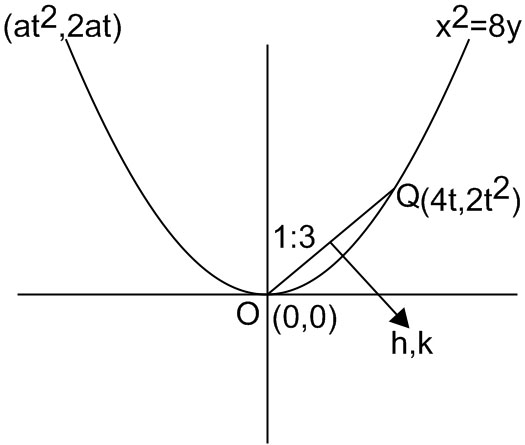#### Let O be the vertex and Q be any point on the parabola, x2 = 8y. If the point Pdivides the line segment OQ internally in the ratio 1 : 3, then the locus of P is : Option 1) x2 = y  Option 2) y2  =  x Option 3) y2  =  2x Option 4) x2 =  2yAs learnt in

Locus -

Path followed by a point p(x,y) under given condition (s).

- wherein

It is satisfied by all the points (x,y) on the locus.

Parametric coordinates of parabola -- wherein

For the parabola.Selection formula -- wherein

If P(x,y) divides the line joining A(x1,y1) and B(x2,y2) in rationParametric coordinatesBy section formulaeliminating t, we get h2 = 2k

=> x2 = 2y

Option 1)

x2 = y

This option is incorrect.

Option 2)

y=  x

This option is incorrect.

Option 3)

y=  2x

This option is incorrect.

Option 4)

x2 =  2y

This option is correct.

##### Posted by